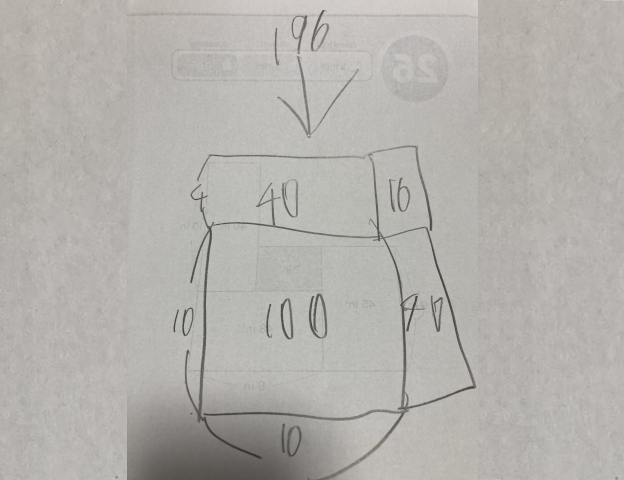A lesson in multiplication opens our eyes to a new way of thinking.

There are a lot of differences between Japan and the Western world, and one of the differences is how children learn to multiply numbers.

In Japan, the method is more visual, and involves a lot more counting rather than straightforward multiplication, which means that once children know how to count, there’s no end to the number of multi-digit numbers they can multiply.

Here to show us how it’s done is a first-year elementary school student, who recently surprised his mother by giving her the correct answer to two-digit multiplication problems like 14 times 14, time and time again.

His mother, who goes by the Twitter handle @shiorinenglish, asked her son to show her how he does it, and so he got out a pencil and paper and showed her this:As you can see, the answer to 14 x 14 is 196. As you can also see, the way he got to that answer is a bit of a head-scratching mystery.

After studying it for a while, though, you can see the logic behind it, and it’s actually a clever approach to a difficult problem.

The numbers being multiplied are first divided into two parts, so in this case, instead of seeing the problem as 14 x 14, you see it as 10 and 4 x 10 and 4. Then, you multiply each of these numbers, which means: 10 x 10, 10 x 4, 4 x 10, and 4 x 4. Then, all that’s left to do is add up the answers, which gives you 196.

Another way to look at it is this:

14×14
=（10+4）×（10+4）
=10×10+4×10+4×10+4×4
=100+40+40+16
=196

Mapping the problem out visually with a diagram like the one above shows you don’t even have to know how to multiply to get the answer, though, as the blocks this son has drawn signify a sum of smaller blocks.

To illustrate:Adding up the four blocks above (100+40+40+16) gives you 196, and that’s exactly what this clever son has illustrated in the photo shared by his proud mum on Twitter.

Some Twitter users likened the young boy’s method for multiplication to the one taught in Numberblocks, a British children’s CGI-animated TV series that also shares clips on its official YouTube channel. This fun, educational series breaks numbers down into blocks, with different characters displaying different block formations that change when added to, subtracted from, or when multiplied or divided.

▼ Changing the way kids “see” numbers, one block at a time.

“Genius!”
“What a wonderful method – I’ll have to remember this!”
“I’d need a calculator for this math problem!”
“Wow, the way he’s drawn it out actually makes it easy to calculate!”
“It just goes to show there’s more than one way to get to the right answer!”

This method really does open our eyes to a whole new way of thinking, and the way this child breaks difficult problems into smaller, more manageable parts is an approach we can apply to all sorts of general problems in life.

So thanks, maths, for once again giving us all the answers to the universe. Now we just need to wrap our heads around these arithmetic problems, which are both right, yet frustratingly wrong in the eyes of Japanese maths teachers.